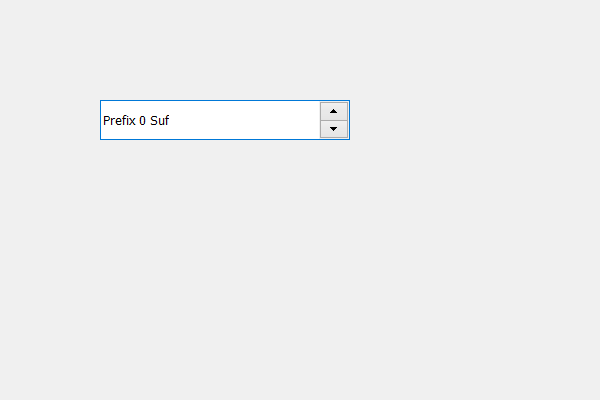Related Articles
PyQt5 QSpinBox – Setting Right Margin
• Last Updated : 19 May, 2020

In this article we will see how we can set or change the right margin of the spin box, by default there is no margin set the to the spin box i.e their margin values are zero. Although we can change that, setting margin to the spin box will not display any change in the spin box due to overriding take place by the widget to maintain its structure. But in order to set margin to the spin box we have set margin to the spin box’s line edit object by which we can display the margin in spin box.

In order to do this we use setRight method.

Syntax : margin.setRight(n)
Here margin is the QMargin object of the QLineEdit object which belongs to spin box

Argument : It take integer as argument

Return : It returns None

Implementation steps :
1. Create a spin box
2. Get the line edit object of the spin box
3. Get the margin object from the spin box
4. Set the right margin to the spin box
5. Add this margin object back to the line edit object

Below is the implementation

 `# importing libraries``from` `PyQt5.QtWidgets ``import` `*` `from` `PyQt5 ``import` `QtCore, QtGui``from` `PyQt5.QtGui ``import` `*` `from` `PyQt5.QtCore ``import` `*` `import` `sys`` ` ` ` `class` `Window(QMainWindow):`` ` `    ``def` `__init__(``self``):``        ``super``().__init__()`` ` `        ``# setting title``        ``self``.setWindowTitle(``"Python "``)`` ` `        ``# setting geometry``        ``self``.setGeometry(``100``, ``100``, ``600``, ``400``)`` ` `        ``# calling method``        ``self``.UiComponents()`` ` `        ``# showing all the widgets``        ``self``.show()`` ` `        ``# method for widgets``    ``def` `UiComponents(``self``):``        ``# creating spin box``        ``self``.spin ``=` `QSpinBox(``self``)`` ` `        ``# setting geometry to spin box``        ``self``.spin.setGeometry(``100``, ``100``, ``250``, ``40``)`` ` `        ``# setting range to the spin box``        ``self``.spin.setRange(``0``, ``999999``)`` ` `        ``# setting prefix to spin``        ``self``.spin.setPrefix(``"Prefix "``)`` ` `        ``# setting suffix to spin``        ``self``.spin.setSuffix(``" Suffix"``)`` ` `        ``# getting line edit object``        ``line_edit ``=` `self``.spin.lineEdit()`` ` `        ``# getting margins``        ``margin ``=` `line_edit.contentsMargins()`` ` `        ``# setting right margin``        ``margin.setRight(``150``)`` ` `        ``# setting this margin back to line edit``        ``line_edit.setContentsMargins(margin)`` ` ` ` ` ` `# create pyqt5 app``App ``=` `QApplication(sys.argv)`` ` `# create the instance of our Window``window ``=` `Window()``window.spin.setFocus()``# start the app``sys.exit(App.``exec``())`

Output :Attention geek! Strengthen your foundations with the Python Programming Foundation Course and learn the basics.

To begin with, your interview preparations Enhance your Data Structures concepts with the Python DS Course. And to begin with your Machine Learning Journey, join the Machine Learning – Basic Level Course

My Personal Notes arrow_drop_up